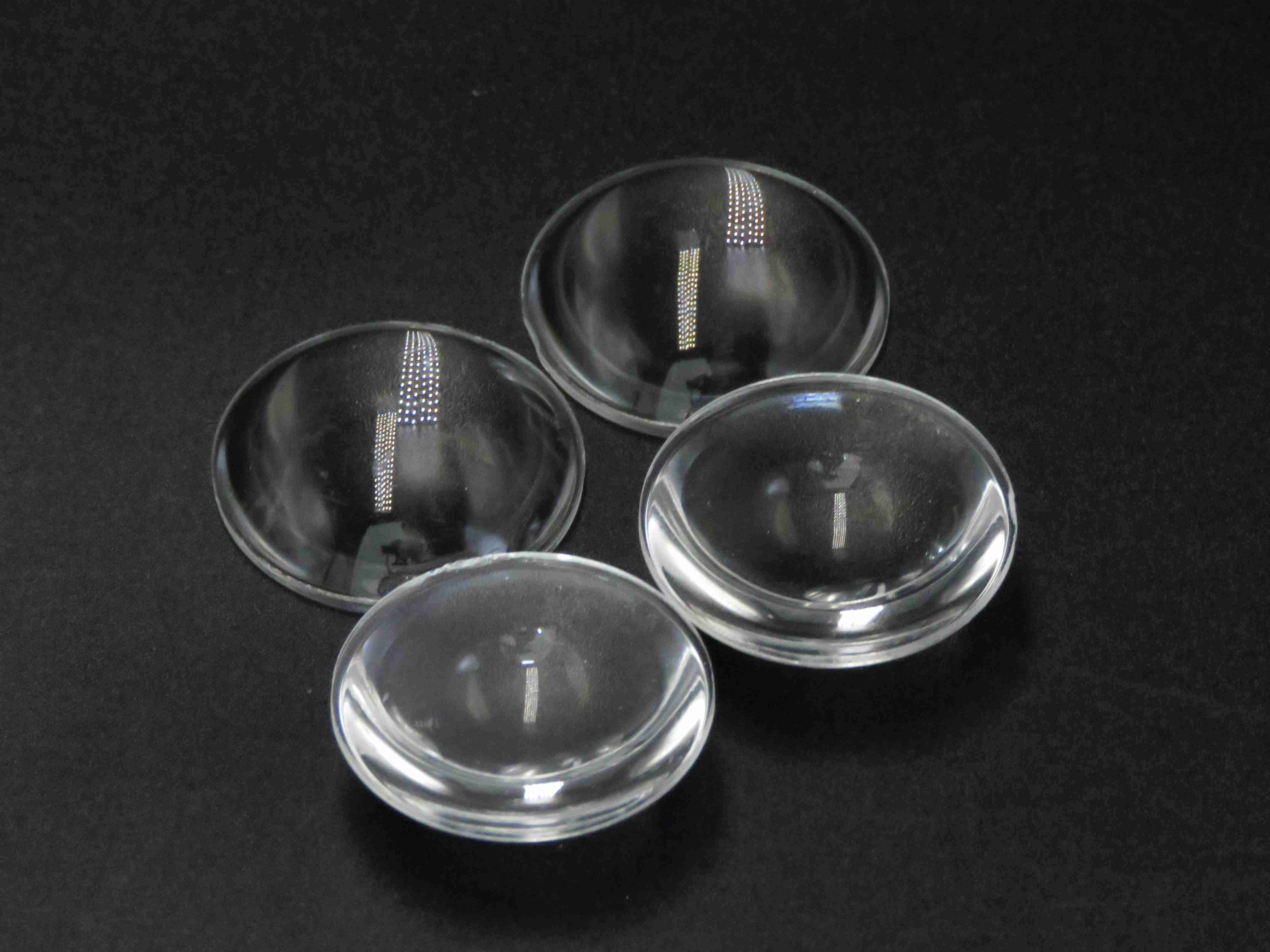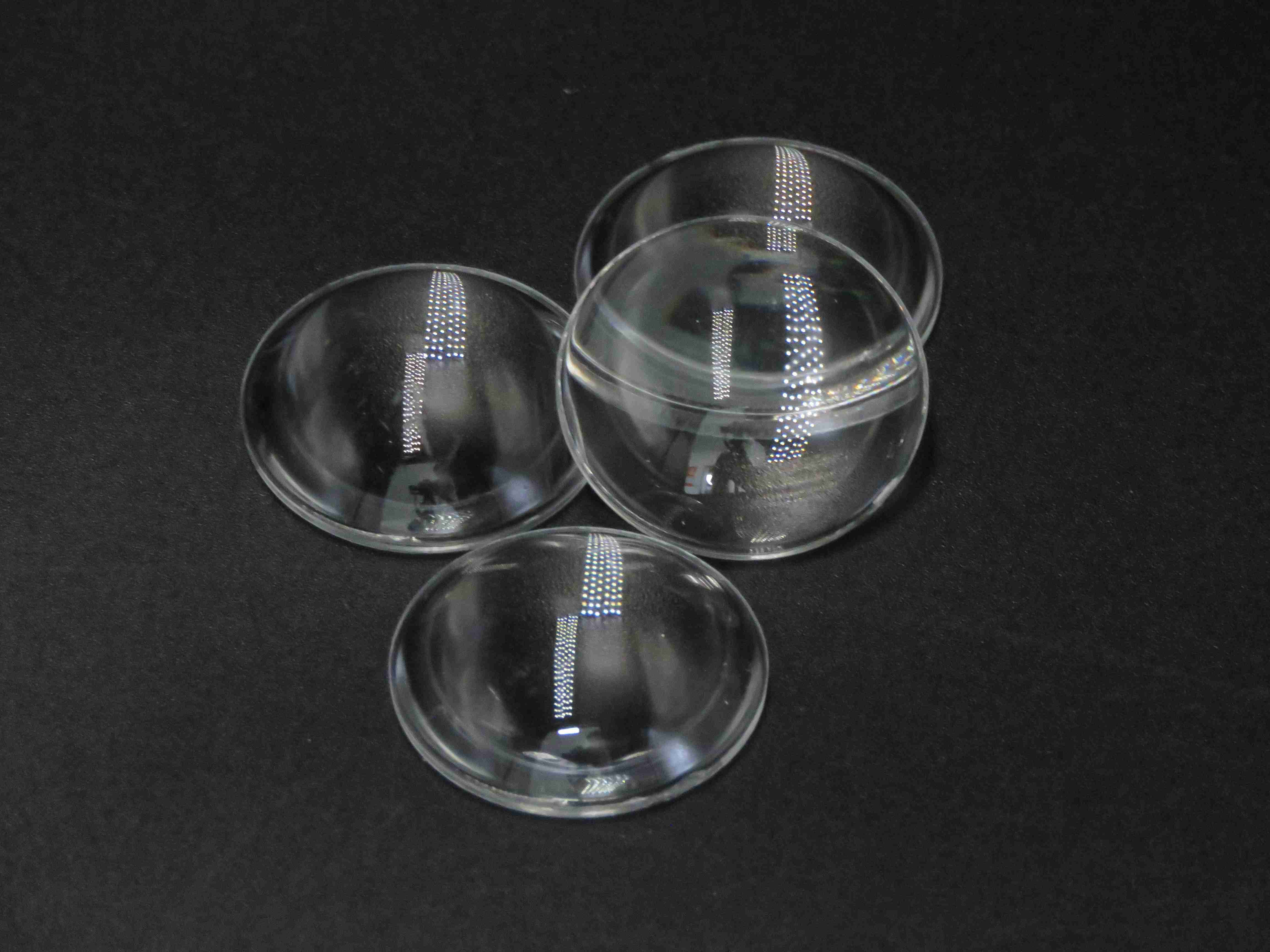# What does the lens include? What is the focal length of a lens?

What does the lens include? What is the focal length of a lens?

A lens is a transparent glass mirror through which light can pass, such as a magnifying glass, reading glasses, and myopia glasses. Because these lenses are different in thickness and size, the focal lengths are different. Divided into real focal length and virtual focal length. A lens is an optical component that refracts light to a certain extent. The focal length of the lens is made according to the requirements and varies from one to the other.

The following editor will share with you what does the lens include? What is the focal length of a lens? content, welcome to read!What does the lens include?

Lenses are classified according to the curvature of two optical surfaces. Both sides of a biconvex lens (or convex lens) protrude. In other words, a lens that is concave on both sides is called a biconcave lens (concave lens). If one surface is flat, it is called a plano-convex or plano-concave lens, determined by the curvature of the other surface. A lens with one surface convex and the other concave is called a convex-concave lens, and if both surfaces have the same curvature, it is called a crescent lens. (In general, crescent lenses generally refer to all forms of convex-concave lenses.

What is the focal length of a lens?

When the parallel light hits the lens, the light is concentrated at a point or the reverse extension line is concentrated at a point, and the distance from this point to the optical center of the lens is the focal length.Focal length is a measure of light gathering or divergence in an optical system, which refers to the distance from the optical center of the lens to the light gathering focal point. It is also the distance of the camera from the center of the lens to the imaging plane such as the film or CCD. A short focal length optical system has better light-gathering ability than a long focal length optical system. Simply put, the focal length is the distance from the focal length to the vertex of the mirror.

For a lens of the same shape, the higher the index of refraction of the material used, the greater the definition by the focal length of the lens.

Thank you for reading, the above is what does the lens include? What is the focal length of a lens? 's introduction. If you want to know more details about lens manufacturers, please contact us and serve you wholeheartedly.